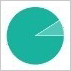[ræd][ræd]• 释义
• 用例
• 词组
• 更多
• n. [物]拉德(吸收辐射剂量单位)### 释义常用度分布图

点击查看

英英释义
Noun:
1. a unit of absorbed ionizing radiation equal to 100 ergs per gram of irradiated material

2. the unit of plane angle adopted under the Systeme International d'Unites; equal to the angle at the center of a circle subtended by an arc equal in length to the radius (approximately 57.295 degrees)

• 例句